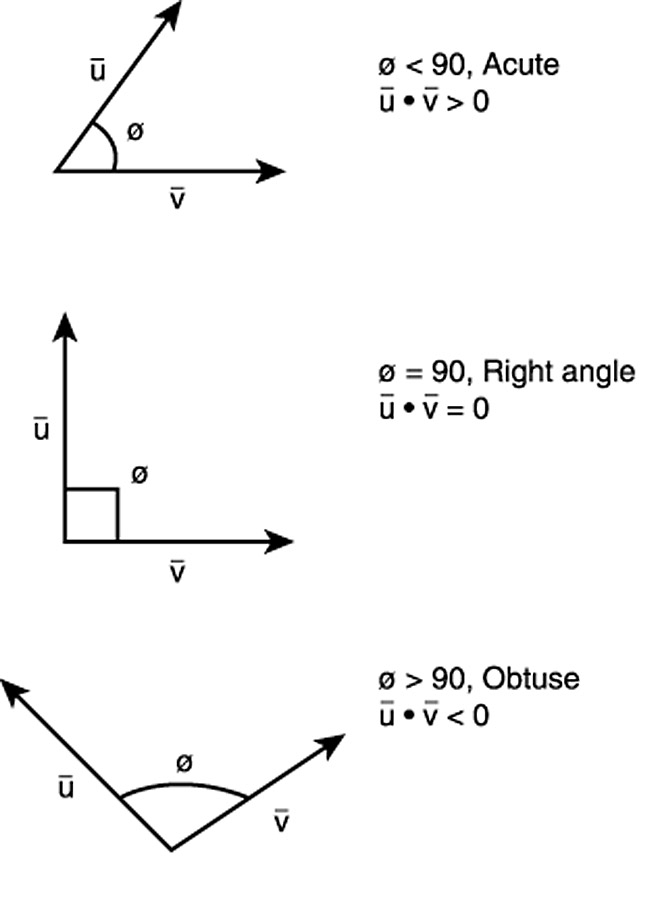## Appendix I – Vector AlgebraVector algebra is formulated to handle vectors; i.e., quantities with both magnitude and direction. Normal algebra, geometry and trigonometry are efficient at dealing with scalar quantities, that is, those with only magnitude, but are inefficient at handling vectors.  Vector algebra is an efficient way of solving 2D and 3D problems…